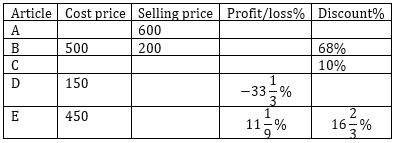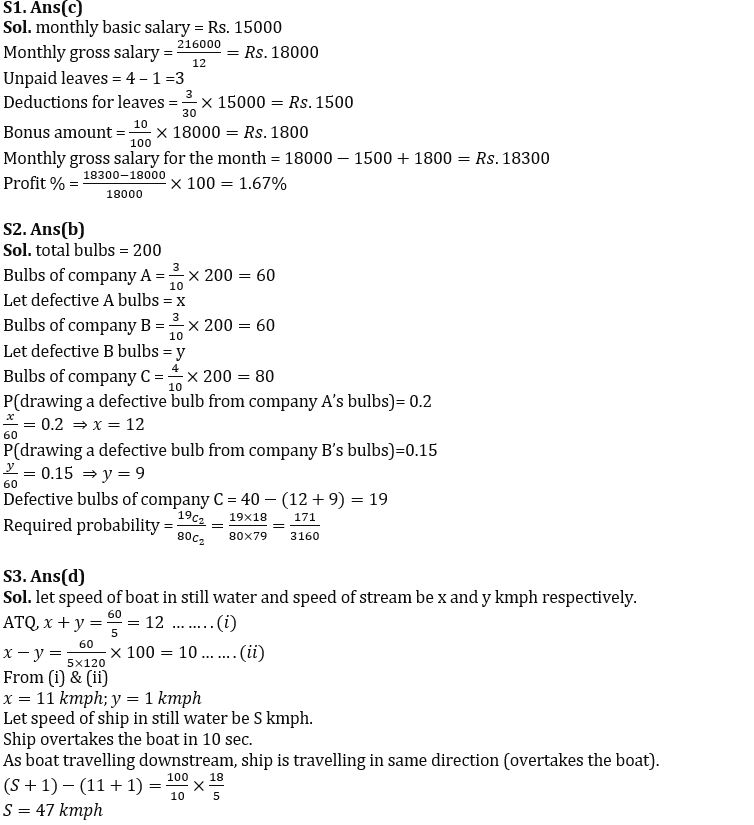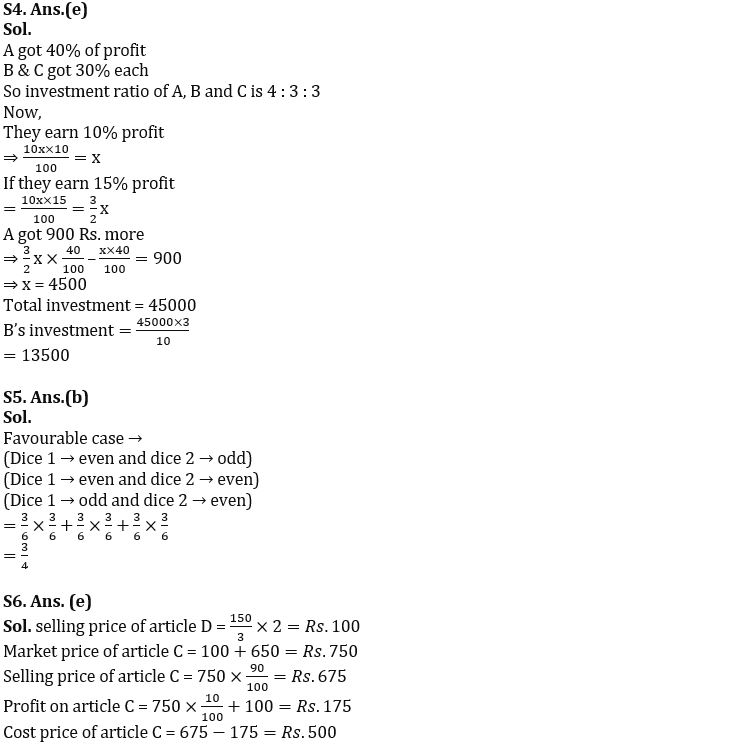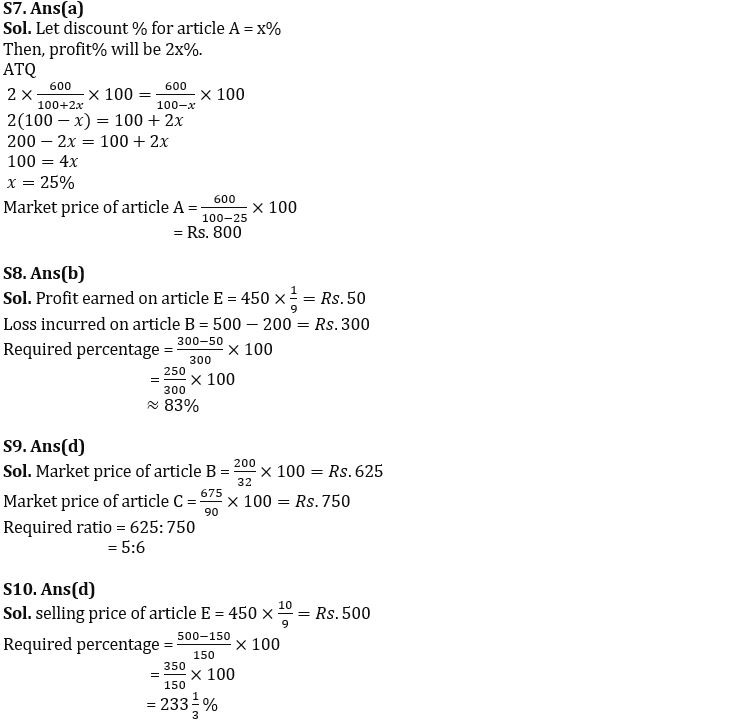Latest Banking jobs   »

# Quantitative Aptitude Quiz For IBPS RRB PO/Clerk Mains 2022- 17th September

Q1. The monthly basic salary of Abhishek is Rs. 15000 while annual gross salary is Rs. 2.16 lakh. He is allowed one paid leave per month after that salary is deducted as per basic salary (on daily basis). In a month, he took 4 leaves but due to his outstanding performance he got 10% of gross monthly salary as bonus. Find his profit/loss percentage on monthly gross salary. (A month is of 30 days)
(a) 1.11% loss
(b) 1.64% profit
(c) 1.67% profit
(d) 1.14% loss
(e) None of these

Q2. In a store, there are total 200 bulb of 3 companies (A, B & C), out of which 40 are defective. Bulbs of company (A, B & C) are in ratio 3:3:4 respectively. Probability of drawing a defective bulb at random from A company’s bulbs and from B company’s bulbs is 0.2 and 0.15 respectively. What is the probability of drawing 2 defective bulbs from C company’s bulbs?
(a) 1/40
(b) 171/3160
(c) 19/80
(d) 57/590
(e) None of these

Q3. A boat covers 60 km in downstream in 5 hours while takes 20% more time in upstream to travel the same distance. On a particular day, the boat while travelling downstream found a ship 100m in length overtakes the boat completely in 10 sec. find the speed of the ship in still water. (in kmph) (ignore length of the boat)
(a) 21
(b) 23
(c) 36
(d) 47
(e) Cannot be determined

Q4. A, B and C entered into a partnership with some investment for one year. After one-year A got 2/5 profit and B and C got equal part of remaining profit. If total profit after one year is 15% instead of 10% then A got 900 Rs. more. Find the investment of B.
(a) 12000
(b) 45000
(c) 27000
(d) 18000
(e) 13500

Q5. Two dice are thrown, find the probability of getting an even number after multiplying numbers obtained from the dice.
(a) ¼
(b) ¾
(c) ⅔
(d) ½
(e) ⅙

Directions (6-10): Table given below gives information about cost price, selling price, profit/loss%(+ve/-ve) and discount % of five different articles, some data are missing in the table calculate the data according to information and answer the following questions.Q6. If for article C profit earned is Rs.100 more than discount given and market price of article C is Rs. 650 more than selling price of article D, find cost price of article C?
(a) Rs. 675
(b) Rs. 525
(c) Rs. 550
(d) Rs. 625
(e) Rs. 500

Q7. If article A is marked 100% above cost price and discount percentage is half of profit percentage, find market price for the same article?
(a) Rs. 800
(b) Rs. 600
(c) Rs. 750
(d) Rs. 1000
(e) Rs. 650

Q8. Profit earned on article E is approximately how much percent less than loss incurred on article B?
(a) 66%
(b) 83%
(c) 111%
(d) 133%
(e) 87%

Q9. What is the ratio of market price of article B to market price of article C, if selling price of article C is Rs.675?
(a) 2:3
(b) 1:1
(c) 4:5
(d) 5:6
(e) None of these.

Q10. Selling price of E is how much percent more than cost price of article D?
(a) 200%
(b) 150%
(c) 166⅔%
(d) 233⅓%
(e) None of these.

Solutions#### Congratulations!Download Hindu Review of October 2021: Free PDF